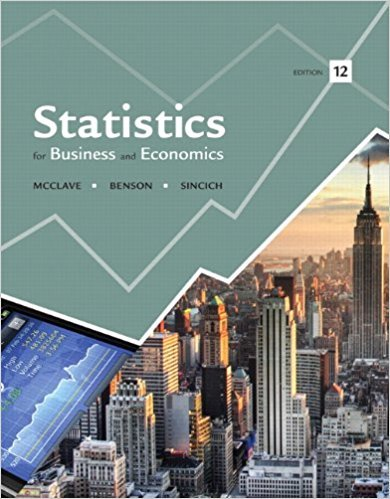×
Log in to StudySoup
Get Full Access to Statistics For Business And Economics - 12 Edition - Chapter 3 - Problem 3e
Join StudySoup for FREE
Get Full Access to Statistics For Business And Economics - 12 Edition - Chapter 3 - Problem 3e

Already have an account? Login here
×
Reset your password

# The sample space for an experiment contains five sampleISBN: 9780321826237 51

## Solution for problem 3E Chapter 3

Statistics for Business and Economics | 12th Edition

• Textbook Solutions
• 2901 Step-by-step solutions solved by professors and subject experts
• Get 24/7 help from StudySoup virtual teaching assistantsStatistics for Business and Economics | 12th Edition

4 5 1 271 Reviews
15
1
Problem 3E

Problem 3E

The sample space for an experiment contains five sample points with probabilities as shown in the table. Find the probability of each of the following events:

 Sample Points Probabilities 1 0.05 2 0.20 3 0.30 4 0.30 5 0.15

A: {Either 1, 2, or 3 occurs}

B: {Either 1, 3, or 5 occurs}

C: {4 does not occur}

Step-by-Step Solution:

Solution

Step 1 of 1

We have to find the probabilities of the given events

Here given that

 Sample point 1 2 3 4 5 Probability 0.05 0.2 0.3 0.3 0.15

A:{Either 1, 2 or 3 occurs}

Then P(A)=P(1)+P(2)+P(3)

=0.05+0.2+0.3

=0.55

B:{Either 1, 3 or 5 occurs}

Then P(B)=P(1)+P(3)+P(5)

=0.05+0.3+0.15

=0.5

C:{4 does not occur}

Then  P(C)=P(1)+P(2)+P(3)+P(5)

=0.05+0.2+0.3+0.15

=0.7

Hence P(A)=0.55,  P(B)=0.5   and P(C)=0.7

Step 2 of 1

##### ISBN: 9780321826237

The answer to “The sample space for an experiment contains five sample points with probabilities as shown in the table. Find the probability of each of the following events:Sample PointsProbabilities10.0520.2030.3040.3050.15A: {Either 1, 2, or 3 occurs}B: {Either 1, 3, or 5 occurs}C: {4 does not occur}” is broken down into a number of easy to follow steps, and 43 words. Statistics for Business and Economics was written by and is associated to the ISBN: 9780321826237. This full solution covers the following key subjects: sample, points, either, Probabilities, occurs. This expansive textbook survival guide covers 15 chapters, and 1631 solutions. The full step-by-step solution to problem: 3E from chapter: 3 was answered by , our top Business solution expert on 07/21/17, 05:42AM. This textbook survival guide was created for the textbook: Statistics for Business and Economics , edition: 12. Since the solution to 3E from 3 chapter was answered, more than 286 students have viewed the full step-by-step answer.

Unlock Textbook Solution

Enter your email below to unlock your verified solution to:

The sample space for an experiment contains five sample